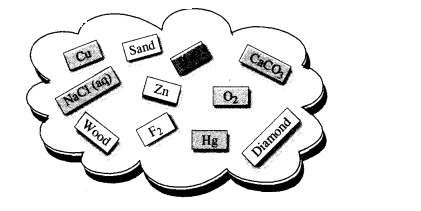# Classify the substances given in the figure into elements and compounds.`
Question:

Classify the substances given in the figure into elements and compounds.Solution:

Elements : $\mathrm{Cu}, \mathrm{Zn}, \mathrm{Hg}$, Diamond.

Compounds: $\mathrm{H}_{2} \mathrm{O}, \mathrm{CaCO}_{3}, \mathrm{O}_{2}, \mathrm{~F}_{2}$

Note: Sand, $\mathrm{NaCl}(\mathrm{aq})$ and wood are the examples of mixtures.